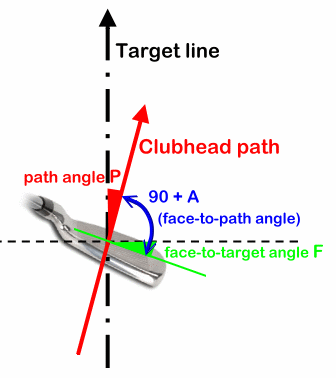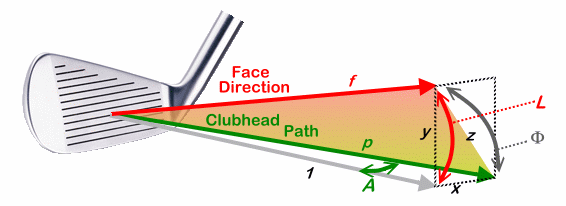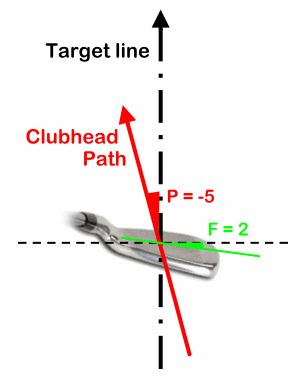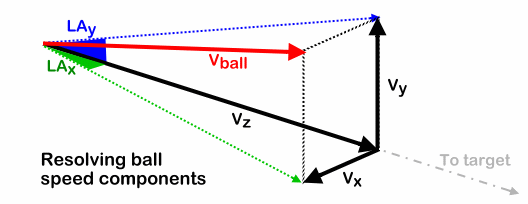3-Dimensional Launch Conditions from Impact Conditions

Dave Tutelman -- March 6, 2007

Going from impact conditions to launch conditions is a challenge. It's even harder in 3D. Here's my cut at how to do it.
(This work was done in support of the development of TrajectoWare Drive. I'd like to thank Frank Schmidberger for his review and corrections.)

## Introduction

In my Club Design Notes, I show how to compute launch conditions from impact conditions in the vertical direction. This 2-dimensional model does not account for side-to-side isues like outside-to-in clubhead path or a closed clubface. So pushes, pulls, slices, fades, hooks and draws are simply not covered. This article fills the gap, and takes the calculations to three dimensions.

Let me start by saying it isn't as simple as applying the same equations in the x-plane that were applied before in the y-plane. The most important difference is that ball speed, launch angle, and spin are not independent in x and y.  They depend on the overall angle in space between the clubface direction and the clubhead path. That angle of overall obliqueness, which we will call Φ, controls most of the process of finding 3D launch conditions.

Here are the steps you have to go through to get the conditions.

## Defining horizontal impact conditions

Vertical impact conditions are well known. They are things like clubhead loft and mass, angle of attack, and clubhead speed. Some of them (clubhead mass and speed) also figure into horizontal impact. But there are horizontal motions to account for, too. We need the equivalents of loft and angle of attack.The figure shows the raw input. It suggests that three angles are important:
• The clubhead path angle P, which we are taking as the angle to the right of the target line. If the path were to the left of the target line, then P would be negative.
• The face-to-path angle A. This is analogous to loft in the vertical direction. It describes the horizontal obliqueness of the hit.
• The face-to-target angle F, which we are defining with respect to the target line. It isn't especially important to the dynamics, but the notion of the face "open" or "closed" is frequently specified with respect to the target line instead of the clubhead path.
The clubhead path P is analogous to angle of attack, and the face-to-path angle A is analogous to loft. If the "face angle" is specified as F rather than A, you can find A for the analysis from:
 A = F - P
So A is a measure of how different the face is from the path. That makes sense. If A is positive, then it gives a clockwise spin (when viewed from above); negative gives a counter-clockwise spin.

So the basic horizontal impact conditions are P and A, even though it's more usual to think of P and F.

## Finding the effective angle of obliqueness

The obvious -- but wrong -- way to use that information is to treat horizontal and vertical conditions the same. That is, use the equations to go from impact conditions to launch conditions independently for horizontal and vertical.

The best argument that this doesn't work is the launch angle. The ball leaves the clubface somewhere between the direction of the clubface and the path of the clubhead. Moreover, its direction is closer to the clubface than the path. But that proportion varies with the obliqueness. For instance, if the obliqueness is 5°, then the proportion is 92%-8%. But if the obliqueness is 20°, then the proportion is 78%-22%. So consider an impact with a loft of 20° and a horizontal obliqueness of 5°. If they could be treated independently, then you would get an upwards launch angle of 15.6° (78% of 20°), but a horizontal launch only 0.4° from the face direction (8% of 5°). That is not right, because the total obliqueness of the impact controls the proportion in both directions, and the total obliqueness is even more than 20°. So you'd need to use a more even distribution than even 78%-22% for both horizontal and vertical launch.

So we have to find the effective obliqueness of the strike, factoring in both loft and face angle. Jorgensen (in his book, "The Physics Of Golf") shows that the launch is somewhere in the plane defined by the two vectors:
• The direction the clubface is facing, and
• The direction the clubhead is moving.
Jorgensen referred to this as "the D-plane", and it is the single most important concept to understand when considering three-dimensional impact. We will call it that when we need to in this discussion.

The key directions are shown in the diagram below, as the red and the green vectors respectively. The shaded plane is Jorgensen's D-plane, the plane of ball direction.Figure 2

L
is the dynamic loft (that is, the loft taking into account thing like shaft bend and clubhead path). The clubface strikes the ball a glancing blow, with A the side angle. The ball rolls up the face in the plane of the shaded triangle, so the effective obliqueness of the strike is Φ. That is what we have to compute.

The gray vector is a "base direction". It is a combination of projections:
• The vertical projection of the face direction, so the angle from the gray vector to the face direction is the dynamic loft L.
• The horizontal projection of the clubhead path, so the angle from the gray vector to the path is the face angle A.
Let's use this information to compute Φ. Here is a diagram labeling the triangles we will use to solve the angle.Figure 3

First let us construct a triangle xyz, in a plane perpendicular to the gray vector. The hypotenuse is part of a triangle fpz, whose vertex at the clubface is the angle Φ that we want to find. Since we are only interested in angles, the triangle could be any size at all, as long as it has the proper angles. So let's set the gray vector's magnitude as the unit vector.

Here is the strategy we will use:
1. We will find x, y, and z as parts of the right triangle.
2. We will find f and p as parts of other right triangles.
3. Now we have three sides of triangle fpz, whose vertex angle is Φ. We can use the side-side-side formula to find Φ.
(1) Find the sides of triangle xyz.
Because of the gray unit vector, we can easily see that:

 x  =  tan(A) y  =  tan(L)

The Pythagorean Theorem tells us that:

 z2  =  x2 + y2  =  tan2(A) + tan2(L)
(2) Find the sides of triangle fpz.
We already have z. We again use the unit vector to find that:

 f  = 1 cos(L)

 p  = 1 cos(A)
(3) Find angle Φ.
The formula for the angle of a side-side-side triangle is well known. For instance, see PlanetMath.org. Applying it to triangle fpz:

cos(Φ) =
 p2 + f2 – z2  2pf

=
 ( 1 cos2(L) + 1 cos2(A) – tan2(L) – tan2(A) ) cos(A) cos(L) 2

=
 ( 1 cos2(L) + 1 cos2(A) – sin2(L) cos2(L) – sin2(A) cos2(A) ) cos(A) cos(L) 2
because
tan(x) = sin(x)/cos(x)

=
 ( 1 - sin2(L) cos2(L) + 1 - sin2(A) cos2(A) ) cos(A) cos(L) 2

=
 ( cos2(L) cos2(L) + cos2(A) cos2(A) ) cos(A) cos(L) 2
because
1-sin2(x) = cos2(x)

=
 ( 1 + 1 ) cos(A) cos(L) 2

cos(Φ) = cos(A) cos(L)

So we know how to find the total obliqueness Φ from loft and side angle. Let's look at the properties of this formula:
• Φ is equal to or larger than either the loft or the face angle. We can see that because  cosine can never be greater than 1.
• Unless A is quite large, Φ is essentially equal to the loft. That is because cosine of a small angle is very close to 1. In order for Φ to differ from the loft by more than 1%, A must be at least 8°.
• Φ is symmetrical in loft and launch angle. Each has the same effect on Φ.
• There is a convenient approximation if you want to calculate Φ by hand and don't have trig tables handy. Just use Pythangorean addition; pretend and A and L are sides of a right triangle and Φ is the hypotenuse.
Φ2 ≅ A2 + L2
Justification for this is in the Appendix.
Now let's use Φ to get launch conditions.

## Computing launch conditionsWe will carry a common example through this, so we see the numbers as we go. It is shown in the diagram on the right:
• The long iron has 23° of loft.
• The swing path is right-to-left ("outside in" for a right-handed golfer) by 5°.
• The clubface points 2° to the right of the target, which we can easily compute to be 7° to the right of the clubhead path.
This is obviously a formula for a slice.

The first thing we need to do is compute A, the horizontal angle between face and path.
 A =  F - P  =  2 - (-5)  =  7°
Now, from the loft and the side angle, we can compute the total oblique angle Φ.
 cos(Φ) = cos(A) cos(L) = cos(7°) cos (23°) = .993 * .921 = .914 = cos ( 24° )
So the total oblique angle is 24°, just a bit larger than the loft of the club. This is fairly common unless the horizontal angle A is quite large.

Now that we know Φ, we can use it to calculate the launch angle (vertical and horizontal), the ball speed, and the spin (backspin and sidespin).

### Launch angle

From the reference we know that, in two dimensions, launch angle is given by:
 LaunchAngle = Loft * (0.96 - 0.0071*Loft)
That works for two dimensions. When we go to three dimensions, then the same equation applies in the D-plane, where Loft becomes Φ. So, in the D-plane:
 LaunchAngle = Φ * (0.96 - 0.0071*Φ)
This is really a proportionality factor times the D-plane "loft" Φ. The proportionality factor tells how much of the loft is converted into launch angle. That factor is not a constant, but rather depends on how oblique the strike is. Let's write it that way:
 LaunchAngle = Φ * K(Φ)
where K(Φ) is the proportionality factor. It is immediately obvious looking at the equations that:
 ProportionalityFactor  =  K(Φ)  =  0.96 - 0.0071 Φ
We can use this to resolve the D-plane launch angle into a vertical and a horizontal component (DAy and DAx respectively). Let's go through it with our already-started numerical example.

For our example, Φ=24°, so the proportionality factor is 0.790. From this, we can calculate vertical launch angle (DAy) and horizontal "launch angle" (DAx). The departure angle is the angle that accounts for the obliqueness of the hit, but does not yet factor in the clubhead path angle P nor angle of attack K.

DAy is the loft times the proportionality factor:
DAy  =  0.790 * Loft  =  0.790 * 23°  =  18.2°
We do something similar to compute DAx.
DAx  =  .790 A   =  .790 * 7°  =  5.5°
Finally, to get the actual launch angle -- that is, the angle with respect to a horizontal line towards the target -- we have to add in the direction the clubhead is traveling -- angle of attack and clubhead path:
LAy  =  DAy + K  =  18.2°
LAx  =  DAx + P   =  0.5°
Where K is positive if attack is upwards, and P is positive if the clubhead is moving to the right at impact.

### Ball speed

From the reference we know that, in two dimensions, ball speed is given by:
 Vball  =  Vclubhead 1 + e 1 + m/M cos(loft)
In three dimensions, the modifier of ball speed is obliqueness rather than just loft, so the equation becomes:
 Vball  =  Vclubhead 1 + e 1 + m/M cos(Φ)
Plugging in some numbers (Vclubhead = 80mph, e = 0.83, m = 46g, M = 248g and of course our computed Φ=24°) we get:
 Vball  =  80 1 + 0.83 1 + 46/248 cos(24°)  =   80 * 1.54 * 0.913  =  112.5mph
For further analysis (such as trajectory calculation) it is useful to decompose this into sideways (x), up/down (y), and "downrange" (z, toward the target) components.Figure 5

This diagram shows the relationship of our calculated ball speed (red vector) to the desired components Vx, Vy, and Vz. We have already computed the horizontal and vertical launch angles LAx and LAy. Let's calculate the component vectors.

It is easy to show that:
Vx2 + Vy2 + Vz2  =  Vball2
(This looks like the Pythagorean Theorem in 3 dimensions, and can be proved by applying the regular Pythagorean Theorem twice, once to each of the triangles sharing the green dotted vector -- or, alternatively, once to each of the triangles sharing the blue dotted vector.)

We also know from basic trigonometry that:
Vx  =  Vz tan (LAx)
Vy  =  Vz tan (LAy)
Plug this into the Pythagorean equation above, and we get:
Vball2   =   Vz2  [1 + tan2(LAx) + tan2(LAy)]
Or:
 Vz2   = Vball2  1 + tan2(LAx) + tan2(LAy)
Now we can calculate Vz, because it is expressed in terms that we already know. Then we can go back to the simple trig formulae above and calculate Vx and Vy from Vz.

Let's go ahead and do that for our example problem. First compute Vz:
 Vz2   = Vball2  1 + tan2(LAx) + tan2(LAy) = 112.52  1 + tan2(0.5°) + tan2(18.2°) =  11,420

Vz   =   sqrt (11,571)   =   106.9mph
Now compute Vx and Vy:
Vx  =  Vz tan (LAx)  =  106.9 tan (0.4°)  =  0.7mph
Vy
=  Vz tan (LAy)  =  106.9 tan (18.2°)  =  35.1mph

### Spin

From the reference we know that, in two dimensions, spin S is given by:
S = 160 * Vclubhead * sin (loft)
where S is in rpm and V is in mph.

As we did with launch angle and ball speed, we have to compute the total spin based on the total obliqueness, then allocate the total spin to vertical and horizontal components.
S = 160 * Vclubhead * sin(Φ)
Now we have to assign spin components Sx and Sy to the horizontal and vertical planes. How do we do that? Look back at Figure 3. The spin will be perpendicular to the plane fpz. Therefore, the spin components are going to be in proportion to each other as x is to y in the figure. In other words:
 Sx Sy = x y = tan(A) tan(L)
Let us designate the ratio of x to y as d; that is  d = tan(A) / tan(L). Since this is the same ratio as the spin components:
 Sx Sy =  d       or       Sx  =  d Sy
The components are at right angle to each other, and vector-sum to form the total spin S. So we have a right triangle with sides Sx and Sy and hypotenuse S. From the Pythagorean Theorem we know that:
Sx2 + Sy2  =  S2
Combining the last two equations:
S2  =  Sy2  +  d2Sy2  =  Sy2 (1 + d2)
We can use this to find Sy:
 Sy  = S  sqrt (1 + d2)
By similar reasoning, we find Sx to be:

 Sx  = d S  sqrt (1 + d2)
Let's use the formulae for our example:
S  =  160 * 80 * sin (24°)  =  5206rpm
The loft L is 23° and the face angle A is 7°. So
 d = tan(7°) tan(23°) = 0.289
Therefore:
 Sx  = d S  sqrt (1 + d2) = .289 * 5206  sqrt (1 + .2892) =  1445rpm

 Sy  = S  sqrt (1 + d2) = 5206  sqrt (1 + .2892) =  5000rpm
Since this original work was done, the Trackman has become all but ubiquitous in discussions about launch and ball flight. They describe spin in different terms. Instead of a horizontal and vertical component, they talk about the spin angle and the total spin. That is probably a more true-to-life way of looking at it. Face it, a ball is not going to have one RPM in the vertical plane and a different RPM in the horizontal plane. We thought it was more intuitive to express things in such a way that one spin component was fighting gravity and the other turning the ball left or right. But both are equally productive ways to look at it, if the purpose is to compute a trajectory.

It is easy enough to compute the Trackman quantities. Just go back to the early part of this subsection, and you will see that:
TotalSpin = 160 * Vclubhead * sin(Φ)

 tan (SpinAngle)  = tan(A) tan(L)
In January 2011, I had the opportunity to match our TrajectoWare computations (which are implemented as described in this article) against Trackman measurements. (Well, Trackman measurements and computations; their estimates of impact angles are computed, not measured directly.) This occurred at Jim McLean's headquarters school at the Doral Golf Resort. We had two computers, one running Trackman and the other running TrajectoWare Drive, and watched Jim's son Jon McLean (a Canadian Tour player) hit balls as we did our thing with the computers. ("OK, now hit a high hook.") The results were very consistent with one another.

## Summary

Here are the steps to get the launch conditions in 3D, given impact conditions in 3D

Given the impact conditions:
• Loft L
• Angle of attack K
• Clubhead speed Vclubhead
• Clubhead path angle P
• Clubhead face-to-path angle A (or A computed from face-to-target angle F, by A = F-P)
• Clubhead mass M
• Ball mass m
• Coefficient of restitution e
Find the launch conditions:
• Horizontal and vertical components of the launch angle: LAx and LAy
• Ball speed Vball
• Sidways, upwards, and downrange components of the ball velocity: Vx, Vy, and Vz
• Horizontal and vertical components of the spin: Sx and Sy
Steps:

(1) Find total obliqueness from
 cos(Φ) = cos(A) cos(L)
(2) Find launch angle with resect to the path from:
DAx  =  A (0.96 - 0.0071 Φ)
DAy  =  L (0.96 - 0.0071 Φ)
(3) Find the launch angles with respect to the target line from:
LAy  =  DAy + K
LAx  =  DAx + P
(4) Find the ball speed from:
 Vball  =  Vclubhead 1 + e 1 + m/M cos(Φ)
(5) Find the components of the ball speed from:
 Vz2   = Vball2  1 + tan2(LAx) + tan2(LAy)
Vx  =  Vz tan (LAx)
Vy  =  Vz tan (LAy)

(6) Find the magnitude of the total spin from:
S = 160 * Vclubhead * sin(Φ)
(7) Find the components of the spin from:
d = tan(A) / tan(L)

 Sy  = S  sqrt (1 + d2)

Sx  =  d Sy

Last modified  March 25, 2017